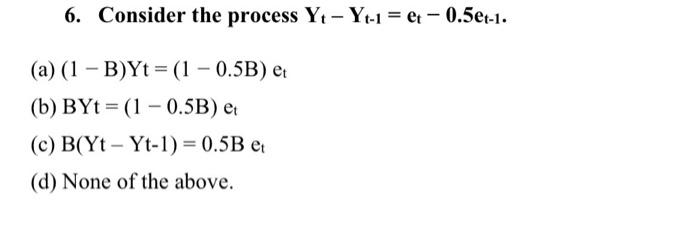# Question 6. Consider the process Yt - Yt-1 = et - 0.5et-1. (a) (1-B)Yt= (1 - 0.5B) et (b) BYt=(1-0.5B) et (c) B(Yt- Yt-1) = 0.5B et (d) None of the above. 6. Consider the process Yt - Yt-1 = et - 0.5et-1. (a) (1-B)Yt= (1 - 0.5B) et (b) BYt=(1-0.5B) et (c) B(Yt- Yt-1) = 0.5B et (d) None of the above.NUG6K2 The Asker · Probability and StatisticsTranscribed Image Text: 6. Consider the process Yt - Yt-1 = et - 0.5et-1. (a) (1-B)Yt= (1 - 0.5B) et (b) BYt=(1-0.5B) et (c) B(Yt- Yt-1) = 0.5B et (d) None of the above.
More
Transcribed Image Text: 6. Consider the process Yt - Yt-1 = et - 0.5et-1. (a) (1-B)Yt= (1 - 0.5B) et (b) BYt=(1-0.5B) et (c) B(Yt- Yt-1) = 0.5B et (d) None of the above.